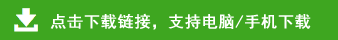# 小学一年级数学上册期末试卷（5套）

小学一年级数学上册期末试卷（5套）部分内容预览

2、画画填填。
■■■■■■■■■＿＿＿      9+（    ）=10
△△△＿＿＿＿＿＿＿＿  3+（    ）=9
○○○○○＿＿＿＿＿＿     5+（    ）=8
3、  6前面一个数是（      ），后面一个数是（      ）。
4、  和8相邻的两个数是（      ）和（       ）。
5、  比5大比10小的数有（                       ）。
6、  ○○○○○○○○○○　  ○有（    ）个，△有（    ）个。
△△△△△△△     ○比△多（  ）个，△比○少（   ）个。
7、
8、在○里填＜、＞或=
10○8            6○6
8○6+1          4-4○0
5+4○10       10-3○8
7-3○2+3      6+3○7+3
9、在□里填上合适的数
□＞9          6+３＜□
9-２＞□      7＜□＜10
□＞8＞□     6+□=10-2
8+□=8－□
10、写出四道得数是5的算式：
□○□=□      □○□=□
□○□=□      □○□=□

4+2=    9-3=      4+5=      6-3=      7+0=
4-4=     6+4=     7-6=      10-5=     2+8=
7+3=    4+4=     3+5=     0+9=      10-8=
8-4=     7-2=      5+5=     10-2=      6-1=

（＿＿＿＿＿  ＿＿＿＿＿）（＿＿＿＿＿　＿＿＿＿＿）

1、大的画“√”，小的画“○”。
2、高大的画“√”，矮的画“○”。
3、○△★○△★○△★○--  下面应画哪一个图形，在正确答案的□里打√。
○    △     ★
□    □     □